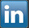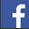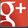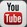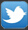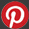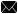info@dolphinmarines.com
Berthing Energy

·  The designed energy to be absorbed by the fender can be calculated as:

Ed = 0.5 x M x VB2 x CM x CE x CC x CS

Where:
Ed = Design energy (under normal conditions) to be absorbed by fender system (in KN)
M = Mass of design vessel (displacement in tones), at chosen confidence level, Usually 95% confidence level
VB = Approach velocity of the vessel perpendicular to the berthing line (m/s).
CM = Added mass coeffi cient
CE = Eccentricity factor
CC = Berth configuration configuration factor or cushion factor
CS = Softness factor

M:  Water displacement of the berthing ship (Tons, Kg, Lbs). - This is the Total Displacement Tonnage(DPT) of the vessel. If you do not have this information you may use our tables to view standard vessel's information by type and sizes.

VB: Berthing velocity of the Ship at the movement of impact against the fender (m/sec, ft/sec) - Berthing velocity is an important parameter in fender system design. It depends on the size of the vessel, loading condition, port structure, and the ease of difficulty of the approach. Therefore the berthing velocity is preferred to be obtained from actual measurements or relevant existing statistical information. When the actual measured velocity is not available, the most widely used guide to estimate the berthing velovity is the Brolsma table, adopted by BSI, PIANC and other standards. To facilitate the calculations, designers can use tables, graphs or equations shown below.CM
: Virtual mass factor - As a vessel makes contact with the berth and its movement is suddenly stopped by the fenders, the mass of water moving with the vessel adds to the energy possessed by the vessel. This is called "Mass Factor" or "Added Mass Coefficient" and the weight of the water is generally called "Additional Weight". The added mass coefficient makes up for the body of water carried along with the ship as it moves sideways through the water. As the vessel is berthing a body of water is carried along with the ship as it moves sideways through the water. As the ship is stopped by the fenders, the momentum of the entrained water continues to push against the ship and this effectively increases its overall mass. CM is normally calculated with the following formula:

CM = 1 + 2 x D/B
where,

Another calculation method for the virtual mass factor is:
CM = π x D2 x L x ρ / (4 xWD)
where,
L: Ship Length
ρ: Sea Water Density(1.025 t/m3)

CE: Eccentricity factor - In the case when a vessel contacts a berth at a point near its bow or stern, the reaction force with give a rotational movement, which will dissipate a part of the vessel's energy.

To determine the Eccentricity Coefficient, you must firstly calculate the radius of gyration(K), the distance from the vessels center of mass to point of impact(R), the velocity vector angle and berthing angle using the following formulas:

CE = [K2 + (R2 x Cos2r)] / (K2 x R2)K = (0.19xCB) + 0.11) x LBP

Where:
K: Radius of rotation of the vessel (usually 1/4 of the vessel's length)
R: Distance of the line paralleled to wharf measured from the vessel's center of gravity to the point of contact. Usually 1/4- 1/5 of vessel's length.
CB: Block Coefficient, which is related to the hull shape and is is calculated as follows:
CB = M / (LBP●B●D●ρSW
Where:
M: Water displacement of the berthing ship(Tons, Kg, Lbs)
ρSW: Sea Water density(1.025 Tons/m3)
LBP: Length between perpendiculars. Please see sketch below for better explanation:x: Distance from bow to point of impact
B: Beam(m, ft)
If the Length, beam and draft are not known, this table can be used to estimate the block coefficient:

 Typical Block Coefficients(CB) Type of Vessel CB CB BS 6349 PIANC 2002 Tankers 0.72~0.85 0.85 Bullk Carriers 0.72~0.85 0.72~0.85 Container Ships 0.65~0.75 0.60~0.80 General Cargo 0.60~0.75 0.72~0.85 RoRo Vessels 0.65~0.70 0.70~0.80 Ferries 0.50~0.65 0.55~0.65

You may also use the following formula to calculate the eccentricity coefficient:
CE = K2/ (K2+R2

Some designers prefer to calculate the eccentricity coefficient using the simplified formula above. Care should be used as this method can lead to an underestimation of Berthing Energy when the berthing angle(α) is greater than 10 degrees and/or the point of impact is aft of quarter-point(x > LBP/4). To verify your calculations, the eccentricity coefficient values generally fall within the following limits:
Quarter-point berthing: x = L/4   Ce = 0.5
Third-point berthing: x = L/3  Ce= 0.6~0.8
Mid-ships berthing: x = L/2  Ce = 1

CC : Berth configuration factor - This is the portion of berthing energy which is absorbed by the cushion effect of water between the approaching vessel and the quay wall. The smaller the draft(D) of the vessel is, or the larger the under keel clearance(KC), the more trapped water can escape under the vessel, and would give a higher CC value.  Also, if the berthing angle of the vessel is greater than 5°, we can consider CC = 1.

Case 1: Closed Dock
A Closed Dock would be a wharf, where you have a concrete wall going directly to the sea ground. In this case the quay wall will push back all the water that is being moved by the vessel. This creates a resistance factor that can be calculated as follows:
If KC ≤  D / 2, CC ≈ 0.8
If KC >  D / 2, CC ≈ 0.9

Case 2: Open or Semi-Closed Dock
A Semi-Closed Dock is a Dock that water can flow underneath the dock, but the depth changes below the dock. Open Dock is usually a dock with piles underneath and the water can flow freely underneath the dock. In such case we can assume the following value of 1.
CC ≈ 1
CS : Softness factor - This is the portion of berthing energy which is absorbed by the deformation of the vessel's hull and fender. When a soft fender is used, CS can be ignored. Otherwise, we can assume a value for CS ≈ 0.9

r = 900 - α - α x Sin[B/(2●R)]
Product Categories
Contact Info
E-mail: info@dolphinmarines.com
Tel: 00852-29738324
Fax: 00852-21159613
Recent Products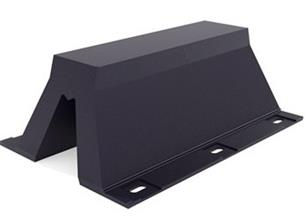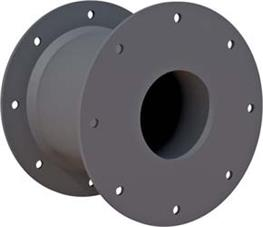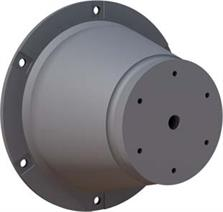Social Networks